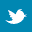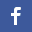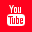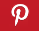Completely Solved C, C++ Programs Assignment.Quick Search Database Videos Tutorials Ebooks Forums FAQ Aboutus Household Industrial Manufacturing Service Shopping Transportation### C program to take a string (full name) and display the initials.

 Filed Under: C Assignments

Problemk Statement:
Write a program to take a string (full name) and display the initials.
Theory:
The first letter of each word of a person’s full name considered as a unit is known as an initial. This program is designed to print the initials of a name entered as a string but it prints the initials of the name along with the surname. Example : Priyanka Sen becomes P.Sen.
Algorithm:
Begin
ba
b’.’
for i0 to less than length of a,increment i
if a[i] is equal to a blank space
b[j]a[i+1]
increment j by 1
b[j]=’.’
Increment j by 1
for ilength of a to a[i] not equal to a blank space,decrement i by 1
set jj-2
for ii+1 to less than length of a,increment i by 1
b[j]a[i]
increment j by 1
set b[j]’\0’
for i0 to less than length of b,increment i by 1
if b[i] is equal to a blank space
increment i by 1
else
print b[i]
End
Program Listing:
//program to print initials of a name
#include<stdio.h>
#include<conio.h>
#include<string.h>
void main()
{
char a;
char b;
int i;
int j=2;
printf(“Enter a name \n”);
gets(a);
b=a;
b=’.’;
for(i=0;i<strlen(a);i++);
{
if(a[i]==’ ‘)
{
b[j]=a[i+1];
j++;
b[j]=’.’;
j++;
}
}
for(i=strlen(a);a[i]!=’ ‘;i--)
j=j-2;
for(i=i+1;i<strlen(a);i++)
{
b[j]=a[i];
j++;
}
b[j]=’\0’;
printf(“The initial is : \n”);
for(i=0;i<strlen(b);i++)
{
if(b[i]==’ ‘)
i++;
else
printf(“%c”,b[i]);
}
}
Output:
Enter a name
Abhisek Basak
The initial is :
A.Basak
Discussion:
• Insertion of a alphabets into the initials string should be alphabets which are preceded by a blank space.
• Keep a check to prevent errors to the output, caused by multiple spaces in between two words while typing the input.Back to main directory:Software Practical